The glass of 1 mm thickness absorb 4% of UV radiation passing through. How many percent of UV rays absorb glass with a thickness 1.1 cm, made from 1 mm thick those glasses?

Result

x =  36.2 %

Solution:Leave us a comment of this math problem and its solution (i.e. if it is still somewhat unclear...):Be the first to comment!To solve this verbal math problem are needed these knowledge from mathematics:

Our percentage calculator will help you quickly calculate various typical tasks with percentages.

Next similar math problems:

1. Piano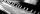If Suzan practicing 10 minutes at Monday; every other day she wants to practice 2 times as much as the previous day, how many hours and minutes will have to practice on Friday?
2. A perineumA perineum string is 10% shorter than its original string. The first string is 24, what is the 9th string or term?
3. A trip to the peak Dry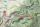Calculate what percentage is rising trip from Strečno to peak Suchý (Dry peak) longer than the descent back. Team trio of tourists (Palo, Peter and Andrew) rise took 4:21 and descent 3:08.
4. JUDr. Usury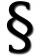Judge JUDr. Usury approved the agreement on guilt and punishment where confessed to pay daily interest 0.18%. How big is a yearly interest? Year has 360 days.
5. Future of libraries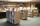You know that thanks to the Internet, electronic communications and developing online resources on the Internet annually declining number of traditional readership by 17%. These deal is irreversible evolutionary shift from old books from libraries to immed
6. Geometric progression 2There is geometric sequence with a1=5.7 and quotient q=-2.5. Calculate a17.
7. Theorem proveWe want to prove the sentence: If the natural number n is divisible by six, then n is divisible by three. From what assumption we started?
8. Six termsFind the first six terms of the sequence a1 = -3, an = 2 * an-1
9. Geometric progression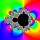Fill 4 numbers between 4 and -12500 to form geometric progression.
10. SequenceFind the common ratio of the sequence -3, -1.5, -0.75, -0.375, -0.1875. Ratio write as decimal number rounded to tenth.
11. GP membersThe geometric sequence has 10 members. The last two members are 2 and -1. Which member is -1/16?
12. Tenth memberCalculate the tenth member of geometric sequence when given: a1=1/2 and q=2
13. Geometric sequence 4It is given geometric sequence a3 = 7 and a12 = 3. Calculate s23 (= sum of the first 23 members of the sequence).
14. Five membersWrite first 5 members geometric sequence and determine whether it is increasing or decreasing: a1 = 3 q = -2
15. Virus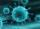We have a virus that lives one hour. Every half hour produce two child viruses. What will be the living population of the virus after 3.5 hours?
16. Effective and mean voltage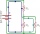A voltage divider consisting of resistors R1 = 103000 Ω and R2 = 197000 Ω is connected to the ideal sine wave voltage source, R2 is connected to a voltmeter which measures the mean voltage and has an internal resistance R3 = 200300 Ω, the measured value is
17. Geometric progression 48,4√2,4,2√2